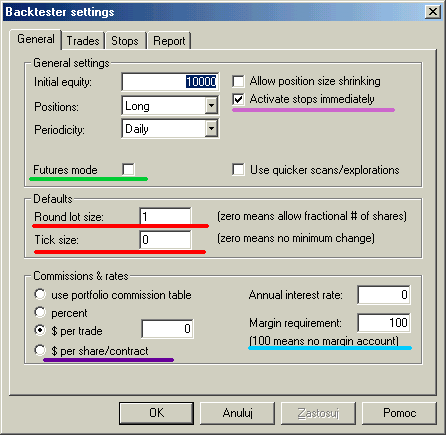## Back-testing systems for futures contracts

Introduction

When you open long position on stocks you just buy given number of shares at given price, then after some time you sell them and your profit is given by difference between sell and buy price mutliplied by number of shares. If you want to open long position on future contract you pay a deposit - margin - for each contract. The margin is just a little part of full contract value (for example 10%). So you can buy 10 contracts paying no more than full value of one contract. This gives you a leverage that makes trading futures more risky than trading stocks. When price of the contract changes your profit/loss changes accordingly. If contract's point value is 1 each 1\$ change in contract price represents 1\$ profit/loss per contract - like in stocks. But futures can have point value different that 1. If, for example, point value is 5 each 1 point change in price of the contract represents 5\$ profit/loss in your equity. When you close position you get the margin deposit back, so your profit/loss is given by number of contracts multiplied by point value mutlipled by difference between sell and buy prices.

Futures mode of the backtester

There are 3 futures-only settings in the backtester:

• Futures mode check box (Settings-General page)
• Margin deposit (Symbol-Information page)
• Point value (Symbol-Information page)Futures mode check box in the settings page (underscored with green line in the picture above) is the key to backtesting futures. It instructs backtester to use margin deposit and point value in calculations.

The remaining settings are per-symbol and they are accessible from Symbol->Information window.Margin deposit

The margin is the amount of money required to open single contract position. You can specify per-symbol margin in the Symbol-Information page (picture above). Positive values describe margin value in dollars, while negative express margin value as percentage of contract price. Margin value of zero is used for stocks (no margin). Margin can be also specified in the formula by using MarginDeposit reserved variable:

`MarginDeposit = 675;`

In the Futures mode margin setting is used to determine how many contacts can be purchased. Let's suppose that your initial equity is set to \$50000 and you want to invest upto 20% of equity in single trade and the margin deposit is \$675. In that case your "desired" position size is 50'000 * 0.2 = 10'000. Provided that you have set round lot size to 1, the backtester will "buy" 10000/675 = (integer)14.8148 = 14 contracts, and true positon value will be \$9450 (18.9% of the initial equity).

To simulate this in AmiBroker you would need to enter 50000 in the Initial Equity field in the backtester, switch on futures mode, and setup remaining parameters in your formula:

```PositionSize = -20; // use 20% of equity MarginDeposit = 675; // this you can set also in the Symbol-Information page RoundLotSize = 1; // this you can set also in the Settings page```

All further trades will use the same logic but position will be sized according to current cumulated equity instead of initial equity level, unless you specify fixed position size in your formula ( PositionSize = 10000 for example).

Point value

Point-value is per-symbol setting (definable in Symbol-Information window - (picture above)) that determines the amount of profit generated by one contract for a one point increase in price. Example: copper is quoted in cents per pound, a price quote of 84.65 (or 8465) equals 84 cents and 65/100 of a cent per pound. A change of +.37 or 37 represents 37/100ths of a cent you will normally hear it quoted as 37 points. But because of the fact that point value for copper is 2.5 every point change gives \$2.5 profit/loss, so in this example profit/loss for the day would be 2.5 * 37 = \$92.50.

You can also set it from the formula level using PointValue reserved variable, for example:

`PointValue = 2.5;`

Note: When you load old database AmiBroker presets point value field to 1 and assumes that by default 1 point represents one dollar so one dollar change gives one dollar profit/loss. This is done to ensure that you get correct results even if you (by mistake) run futures mode test on stocks.

Note 2: Although point value setting affects (multiplies) profits/losses it does NOT affect built-in stops. The stops ALWAYS operate on price movement alone. So you should be aware that setting 10% profit target stop will result in 25% profit on trade exited by this stop when point value is set to 2.5.

Simple cases

Points-only test

Points only test is equivalent to trading just one contract. This can be easily accomplished using Futures mode of the backtester and adding the following one line to your formula:

`PositionSize = MarginDeposit = 1;`

```NumContracts = 7; PositionSize = NumContracts * MarginDeposit;```